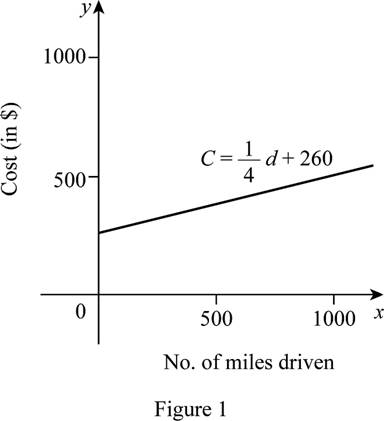# To express: The monthly cost in terms of the distance driven d assuming the function follows a linear function.### Single Variable Calculus: Concepts...

4th Edition
James Stewart
Publisher: Cengage Learning
ISBN: 9781337687805### Single Variable Calculus: Concepts...

4th Edition
James Stewart
Publisher: Cengage Learning
ISBN: 9781337687805

#### Solutions

Chapter 1.2, Problem 18E

(a)

To determine

## To express: The monthly cost in terms of the distance driven d assuming the function follows a linear function.

Expert Solution

The equation of the monthly cost in terms of the distance driven d is C=14d+260.

### Explanation of Solution

Let d represents the number of miles driven in a month and C represents the monthly cost in dollars.

Recall the general equation of the linear function y=mx+c where m is the slope and c is the y-intercept.

Since the cost function follows a linear function, the equation of the cost C in terms of the number of miles driven d is in the form of C=md+c.

According to the given data, there are two points such as (480, 380) and (800, 460).

Obtain the slope m by using the two point formula as follows.

m=y2y1x2x1=460380800480=80320=14

Thus, the slope is m=14.

Use the slope m=14 and any one of the two points say (800, 460) and obtain the equation of cost C in terms of d as follows.

yy1=m(xx1)     [Slope-pointform]C460=14(d800)C=14d8004+460C=14d+260

Thus, the required equation is C=14d+260.

(b)

To determine

Expert Solution

(c)

To determine

Expert Solution

### Explanation of Solution

Let x-axis represents the number of miles driven and y-axis represents the monthly cost in dollars.

From part (a), the equation of the monthly cost as a function of distance driven is C=14d+260.

Obtain the values of C for several values of d as tabulated in Table 1 and draw the graph as shown below in Figure 1.

 d C 0 260 500 385 1000 510

Table 1From Figure 1, it is observed that the graph is a straight line as the function is linear.

Also, notice that the cost increases as the number of miles increases. That is, if the distance driven increases by 320, then the cost increases by $80 ($0.25 per mile.)

Thus, the slope is, m=14 represents the rate of change of cost with respect to the number of miles driven.

(d)

To determine

### To explain: The meaning of C-intercept.

Expert Solution

The C-intercept of the cost function is 260 and it represents the fixed manufacturing cost per day.

### Explanation of Solution

From part (a), the equation of the monthly cost as a function of the distance driven is C=14d+260.

Since it follows a linear function, the constant term c is considered as the y-intercept.

Thus, the y-intercept is 260.

The C-intercept represents the fixed monthly cost as it is the constant term.

(e)

To determine

Expert Solution

### Explanation of Solution

Since the monthly cost is fixed and the cost increases as the distance driven increases, the function follows the linear function.

Thus, the linear function is suitable for this situation.

### Have a homework question?

Subscribe to bartleby learn! Ask subject matter experts 30 homework questions each month. Plus, you’ll have access to millions of step-by-step textbook answers!